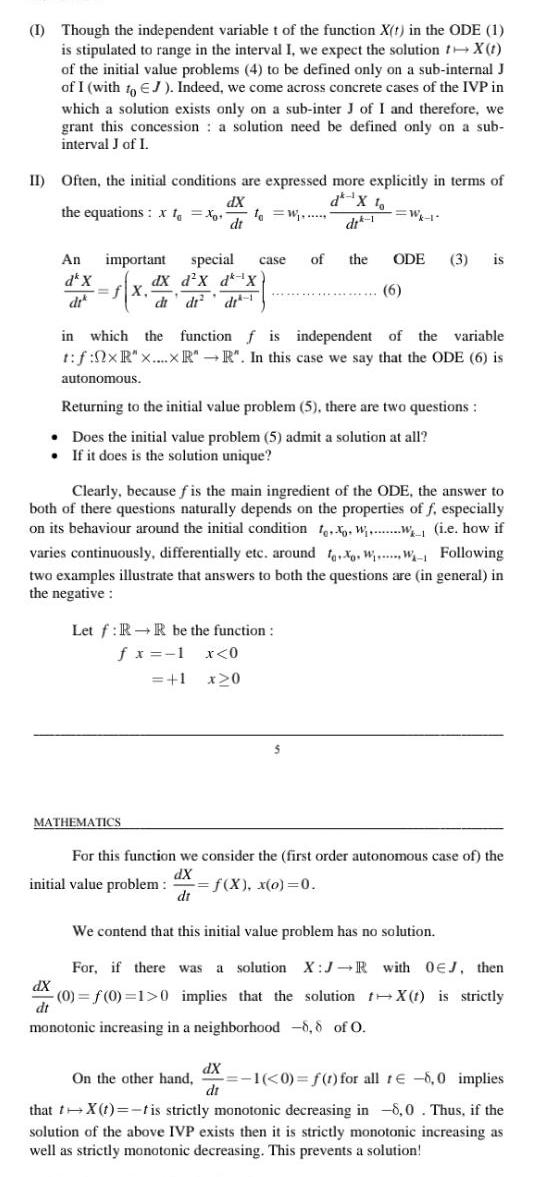Question:

# I Though the independent variable t of the function X t in

Last updated: 9/18/2023I Though the independent variable t of the function X t in the ODE 1 is stipulated to range in the interval I we expect the solution X t of the initial value problems 4 to be defined only on a sub internal J of I with to EJ Indeed we come across concrete cases of the IVP in which a solution exists only on a sub inter J of I and therefore we grant this concession a solution need be defined only on a sub interval J of I II Often the initial conditions are expressed more explicitly in terms of dX the equations X to Xo to W dt An important special fX dX dx d X d X di di di case MATHEMATICS di 1 in which the function f is independent of the variable t f 0xR x xR R In this case we say that the ODE 6 is autonomous Let f RR be the function f x 1 x 0 1 x 0 Returning to the initial value problem 5 there are two questions Does the initial value problem 5 admit a solution at all If it does is the solution unique Clearly because fis the main ingredient of the ODE the answer to both of there questions naturally depends on the properties of f especially on its behaviour around the initial condition to Ww i e how if varies continuously differentially etc around X WW Following two examples illustrate that answers to both the questions are in general in the negative Wk 1 of the ODE 3 is 6 For this function we consider the first order autonomous case of the dX initial value problem f X x o 0 dt dX di We contend that this initial value problem has no solution For if there was a solution X J R with OEJ then 0 f 0 1 0 implies that the solution X t is strictly dt monotonic increasing in a neighborhood 8 8 of O On the other hand 1 0 f t for all re 8 0 implies that t X t t is strictly monotonic decreasing in 8 0 Thus if the solution of the above IVP exists then it is strictly monotonic increasing as well as strictly monotonic decreasing This prevents a solution